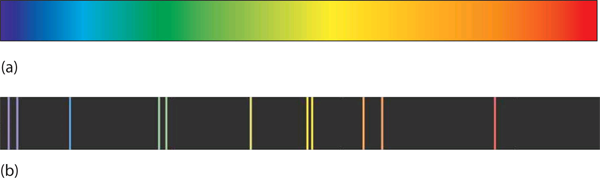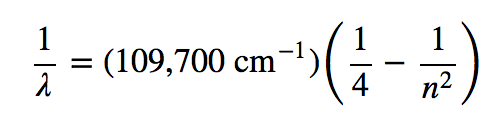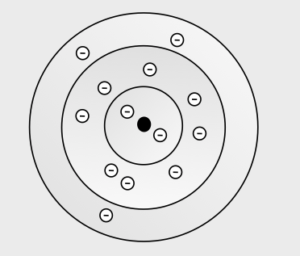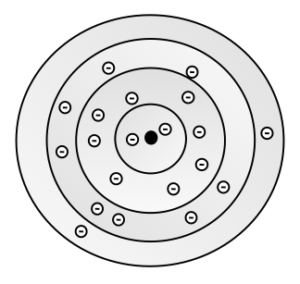Chapter 8. Electronic Structure of Atoms

# 8.2 Quantization of the Energy of Electrons

### Learning Objectives

By the end of this module, you will be able to:

• Explain what spectra are.
• Describe Bohr’s Model of the hydrogen atom.
• Describe the Electron Shell Model.

There are two fundamental ways of generating light: either heat an object up so hot it glows or pass an electrical current through a sample of matter (usually a gas). Incandescent lights and fluorescent lights generate light via these two methods, respectively.

A hot object gives off a continuum of light. We notice this when the visible portion of the electromagnetic spectrum is passed through a prism: the prism separates light into its constituent colors, and all colors are present in a continuous rainbow (part (a) in Figure 1 “Prisms and Light”). This image is known as a continuous spectrum. However, when electricity is passed through a gas and light is emitted and this light is passed though a prism, we see only certain lines of light in the image (part (b) in Figure 1 “Prisms and Light”). This image is called a line spectrum. It turns out that every element has its own unique, characteristic line spectrum.Figure 1. Prisms and Light  (a) A glowing object gives off a full rainbow of colors, which are noticed only when light is passed through a prism to make a continuous spectrum. (b) However, when electricity is passed through a gas, only certain colors of light are emitted. Here are the colors of light in the line spectrum of Hg.

Why does the light emitted from an electrically excited gas have only certain colors, while light given off by hot objects has a continuous spectrum? For a long time, it was not well explained. Particularly simple was the spectrum of hydrogen gas, which could be described easily by an equation; no other element has a spectrum that is so predictable (Figure 2 “Hydrogen Spectrum”).

Late-nineteenth-century scientists found that the positions of the lines obeyed a pattern given by the equationwhere n = 3, 4, 5, 6,…, but they could not explain why this was so.  The spectrum of hydrogen was particularly simple and could be predicted by a simple mathematical expression.

In 1913, the Danish scientist Niels Bohr suggested a reason why the hydrogen atom spectrum looked this way. He suggested that the electron in a hydrogen atom could not have any random energy, having only certain fixed values of energy that were indexed by the number n (the same n in the equation above and now called a quantum number) (Figure 3). Quantities that have certain specific values are called quantized. Bohr suggested that the energy of the electron in hydrogen was quantized because it was in a specific orbit. Because the energies of the electron can have only certain values, the changes in energies can have only certain values (somewhat similar to a staircase: not only are the stair steps set at specific heights but the height between steps is fixed).

Finally, Bohr suggested that the energy of light emitted from electrified hydrogen gas was equal to the energy difference of the electron’s energy states:

Elight = hν = ΔEelectron

This means that only certain frequencies (and thus, certain wavelengths) of light are emitted. Figure 4 “Bohr’s Model of the Hydrogen Atom” shows a model of the hydrogen atom based on Bohr’s ideas.

Bohr’s description of the hydrogen atom had specific orbits for the electron, which had quantized energies.

Postulates of the Bohr Model:

1)  Electrons move in specific circular orbits only.
2)  As an atom absorbs energy, the electron jumps to a larger orbit, of higher energy (an excited state).
3)  As an atom emits energy, it “falls” to a smaller, lower energy orbit.

This model represented a great intellectual achievement by Bohr, as it was the first atom model that invoked quantization of the electron energy in some way. Also his mathematical formula  which calculated the energy of the electron in any orbit, matched the real energies observed in experiments with hydrogen. However, the theory had significant limitations.

Some Key Problems with the Bohr Model:

• It only works for hydrogen (though can be adapted to other one electron ions). If there are 2 or more electrons, the mathematical formula does not match real data.
• It is fundamentally incorrect in that electrons do not move in fixed orbits!

## The Electron Shell Model of the Atom

We can overcome one of the key objections to the Bohr Model by abandoning the concept of electrons moving in fixed diameter orbits. Instead we envision a series of spherical shells of increasing size surrounding the nucleus in which the electrons reside (Figure 5). The Electron Shell Model does not attempt to describe the movement of the electrons, only that each shell has a different size and energy and the electron moves within that space. The quantum jumps of the electron are thus the electron moving from one shell to another.

We also account for other experimental evidence and specify that the shells can hold a certain maximum number of electrons. Table 1 shows this maximum filling, as well as some other aspects of these shells.

## The Electron Configuration of Atoms using the Shell Model

So, for a given atom or ion, in which shell(s) do the electrons reside? It turns out the electrons follow a simple principle, namely, they go into the lowest energy shell that is available. If a lower energy shell is full, they go into the next lowest energy shell. A crude analogy is putting water into a pail; the water always fills from the bottom! So to establish this electron configuration, first determine the number of electrons the atom has, then “put” them into the shells as the above rule dictates. Look at Figure 5 again, which represents an atom with 13 electrons. Notice how the lower energy shells are full, and the last three electrons go into shell 3, which is not full. Additional electrons would continue to go into shell 3 until it is full with 8 electrons, for a total of 18. A 19th electron would be forced to go into shell 4.

### Example 1

Draw an electron shell model of an aluminum atom.

Solution

Step 1: Determine the number of electrons.

Since it is not specified that the atom is charged, we presume it is neutral. Aluminum has 13 protons, so neutral aluminum would have 13 electrons.

Step 2: Determine the electron configuration.

Put 2 electrons in shell 1 which fills it, next put 8 electrons in shell 2 which fills it, and the last three electrons go into shell 3.

Step 3: Draw the image.Test Yourself

Draw an electron shell model of a calcium atom.## Electron Configurations and the Periodic Table

Look at the number of elements in each row of the periodic table. Rows 1 through 4 contain 2, 8, 8, and 18 elements respectively. Now look at Table 1. Is this a coincidence? No! In fact this shows that the patterns of elemental properties that the periodic table reflects have their basis in electron configurations. Consider Figure 6 which shows the electron shell models of hydrogen, lithium, sodium, and potassium.

See how each has one electron in its highest energy shell. Now find these elements on the periodic table. They are all in the first column of the periodic table. Consider the elements of the last column of the periodic table (draw them out for yourself). They all have full outer shells. A general relationship begins to emerge: elements in the same column on the periodic table have similar electron configurations.

Originally, the periodic table was constructed based on observable chemical and physical properties. Elements that behaved similarly were placed in the same column; however the chemists had no explanation of why they were similar. Now with the electron shell model we have a theory that helps us understand the reasons for these similarities.

### Chemistry Is Everywhere: Neon Lights

A neon light is basically an electrified tube with a small amount of gas in it. Electricity excites electrons in the gas atoms, which then give off light as the electrons go back into a lower energy state. However, many so-called “neon” lights don’t contain neon!

Although we know now that a gas discharge gives off only certain colors of light, without a prism or other component to separate the individual light colors, we see a composite of all the colors emitted. It is not unusual for a certain color to predominate. True neon lights, with neon gas in them, have a reddish-orange light due to the large amount of red-, orange-, and yellow-colored light emitted. However, if you use krypton instead of neon, you get a whitish light, while using argon yields a blue-purple light. A light filled with nitrogen gas glows purple, as does a helium lamp. Other gases—and mixtures of gases—emit other colors of light. Ironically, despite its importance in the development of modern electronic theory, hydrogen lamps emit little visible light and are rarely used for illumination purposes.

## Key Concepts and Summary

Bohr incorporated Planck’s and Einstein’s quantization ideas into a model of the hydrogen atom that resolved the paradox of atom stability and discrete spectra. The Bohr model of the hydrogen atom explains the connection between the quantization of photons and the quantized emission from atoms. Bohr described the hydrogen atom in terms of an electron moving in a circular orbit about a nucleus. He postulated that the electron was restricted to certain orbits characterized by discrete energies. Transitions between these allowed orbits result in the absorption or emission of photons. When an electron moves from a higher-energy orbit to a more stable one, energy is emitted in the form of a photon. To move an electron from a stable orbit to a more excited one, a photon of energy must be absorbed. Using the Bohr model, we can calculate the energy of an electron and the radius of its orbit in any one-electron system.

### Exercises

1. What does it mean to say that the energy of the electrons in an atom is quantized?

2. How are the Bohr model and the Rutherford model of the atom similar? How are they different?

3. Differentiate between a continuous spectrum and a line spectrum.

1. Quantized energy means that the electrons can possess only certain discrete energy values; values between those quantized values are not permitted.

2. Both involve a relatively heavy nucleus with electrons moving around it, although strictly speaking, the Bohr model works only for one-electron atoms or ions. According to classical mechanics, the Rutherford model predicts a miniature “solar system” with electrons moving about the nucleus in circular or elliptical orbits that are confined to planes. If the requirements of classical electromagnetic theory that electrons in such orbits would emit electromagnetic radiation are ignored, such atoms would be stable, having constant energy and angular momentum, but would not emit any visible light (contrary to observation). If classical electromagnetic theory is applied, then the Rutherford atom would emit electromagnetic radiation of continually increasing frequency (contrary to the observed discrete spectra), thereby losing energy until the atom collapsed in an absurdly short time (contrary to the observed long-term stability of atoms). The Bohr model retains the classical mechanics view of circular orbits confined to planes having constant energy and angular momentum, but restricts these to quantized values dependent on a single quantum number, n. The orbiting electron in Bohr’s model is assumed not to emit any electromagnetic radiation while moving about the nucleus in its stationary orbits, but the atom can emit or absorb electromagnetic radiation when the electron changes from one orbit to another. Because of the quantized orbits, such “quantum jumps” will produce discrete spectra, in agreement with observations.

3. A continuous spectrum is a range of light frequencies or wavelengths; a line spectrum shows only certain frequencies or wavelengths.

## Glossary

Bohr’s model of the hydrogen atom: structural model in which an electron moves around the nucleus only in circular orbits, each with a specific allowed radius; the orbiting electron does not normally emit electromagnetic radiation, but does so when changing from one orbit to another.

excited state: state having an energy greater than the ground-state energy

ground state: state in which the electrons in an atom, ion, or molecule have the lowest energy possible